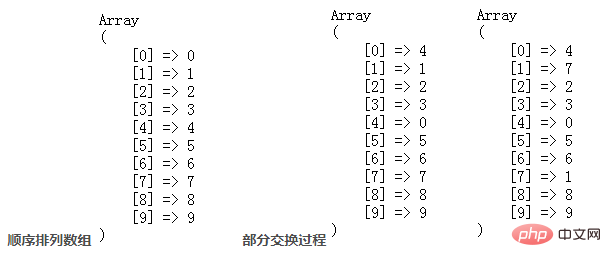# php如何使用rand生成不重复随机数_编程技术_编程开发技术教程

php使用rand生成不重复随机数的方法：首先为数组的每个数字按其下标顺序赋值；然后开始取范围[ i，\$num-1 ]范围内的随机数【\$rand】，并将获取到的随机数【\$rand】作为数组中当前位置；最后在未改变的键值对中进行交替运算。php使用rand生成不重复随机数的方法：

```/*
* array unique_rand( int \$min, int \$max, int \$num )
* 生成一定数量的不重复随机数
* \$min 和 \$max: 指定随机数的范围
* \$num: 指定生成数量
*/
function unique_rand(\$min, \$max, \$num) {
//初始化变量为0
\$count = 0;
//建一个新数组
\$return = array();
while (\$count < \$num) {
//在一定范围内随机生成一个数放入数组中
\$return[] = mt_rand(\$min, \$max);
//去除数组中的重复值用了“翻翻法”，就是用array_flip()把数组的key和value交换两次。这种做法比用 array_unique() 快得多。
\$return = array_flip(array_flip(\$return));
//将数组的数量存入变量count中
\$count = count(\$return);
}
//为数组赋予新的键名
shuffle(\$return);
return \$return;
}```

PHP高效产生m个n范围内的不重复随机数（m<=n)

```function rand_num(\$num='200'){
for(\$i=0;\$i<\$num;\$i++){
\$n[\$i] = \$i;
}
for(\$i=0;\$i<\$num;\$i++){
\$rand = mt_rand(\$i,\$num-1);
//数组  随机数交换下标
if(\$n[\$i] == \$i){
\$n[\$i] = \$n[\$rand];
\$n[\$rand] = \$i;
}
}
}```

1.第一步，为数组的每个数字按其下标顺序赋值，获得一个 \$num 个数字键值对应顺序排列的数组。

2.第二步，开始取范围[ i，\$num-1 ]范围内的随机数\$rand,并将获取到的随机数\$rand作为数组中当前位置 键i对应下标的值\$rand，将数组中 键\$rand对应下标的值替换为 i，这其实就是数组键值交叉互换。意义是，将已经生成的随机数在取值范围[ i,\$num-1 ]中排除，下次会在剩下的数字中[ i+1,num-1 ]取值。

3.第三步，为避免重复取值，只在未改变的键值对中进行交替运算，即在原数组顺序排列 (键==值) 的位置进行交替运算。

4.结束。php如何使用rand生成不重复随机数

php如何设置日志输出

## PHP中如何使用cURL获取JSON数据并解码

PHP中如何使用cURL获取JSON数据并解码

## php怎么设置SESSION_ID

php怎么设置SESSION_ID

php中时间转时间戳的函数是什么